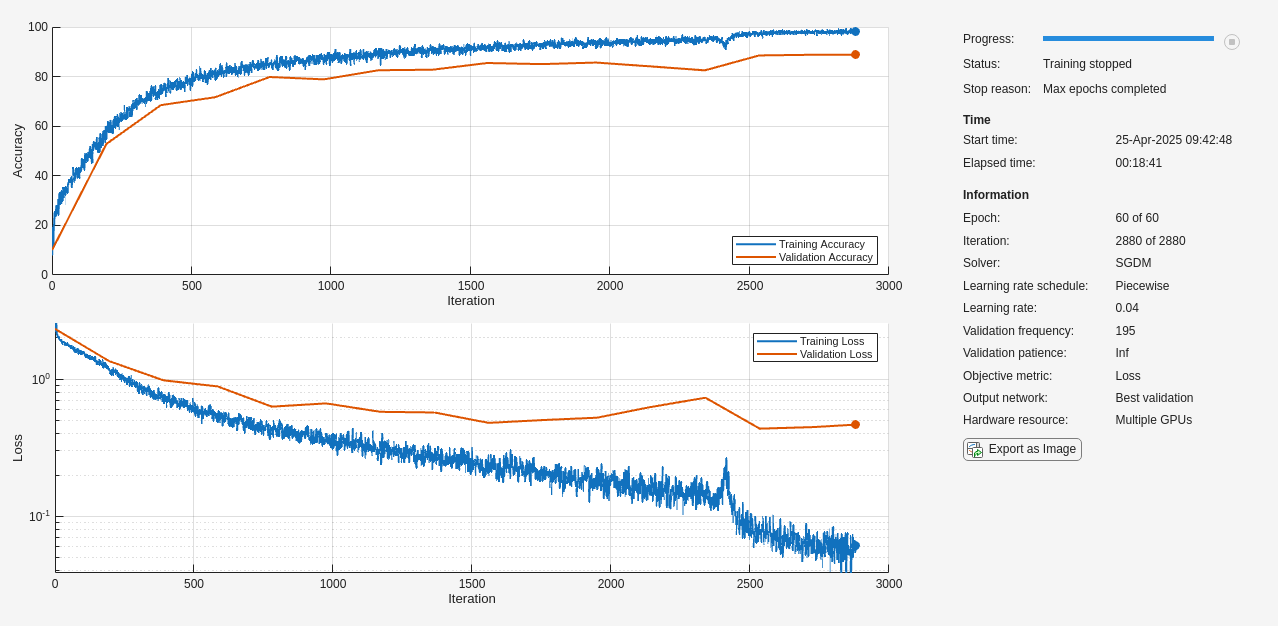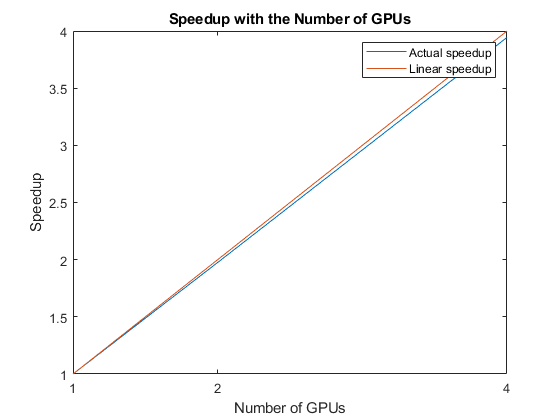# Train Network Using Automatic Multi-GPU Support

This example shows how to use multiple GPUs on your local machine for deep learning training using automatic parallel support.

Training deep learning networks often takes hours or days. With parallel computing, you can speed up training using multiple GPUs. To learn more about options for parallel training, see Scale Up Deep Learning in Parallel, on GPUs, and in the Cloud.

### Requirements

Before you can run this example, you must download the CIFAR-10 data set to your local machine. To download the CIFAR-10 data set, use the `downloadCIFARToFolders` function, attached to this example as a supporting file. To access this file, open the example as a live script. The following code downloads the data set to your current directory. If you already have a local copy of CIFAR-10, then you can skip this section.

```directory = pwd; [locationCifar10Train,locationCifar10Test] = downloadCIFARToFolders(directory);```
```Downloading CIFAR-10 data set...done. Copying CIFAR-10 to folders...done. ```

Load the training and test data sets by using an `imageDatastore` object. In the following code, ensure that the location of the datastores points to CIFAR-10 in your local machine.

```imdsTrain = imageDatastore(locationCifar10Train, ... IncludeSubfolders=true, ... LabelSource="foldernames"); imdsTest = imageDatastore(locationCifar10Test, ... IncludeSubfolders=true, ... LabelSource="foldernames");```

To train the network with augmented image data, create an `augmentedImageDatastore` object. Use random translations and horizontal reflections. Data augmentation helps prevent the network from overfitting and memorizing the exact details of the training images.

```imageSize = [32 32 3]; pixelRange = [-4 4]; imageAugmenter = imageDataAugmenter( ... RandXReflection=true, ... RandXTranslation=pixelRange, ... RandYTranslation=pixelRange); augmentedImdsTrain = augmentedImageDatastore(imageSize,imdsTrain, ... DataAugmentation=imageAugmenter);```

### Define Network Architecture and Training Options

Define a network architecture for the CIFAR-10 data set. To simplify the code, use convolutional blocks that convolve the input. The pooling layers downsample the spatial dimensions.

```blockDepth = 4; % blockDepth controls the depth of a convolutional block. netWidth = 32; % netWidth controls the number of filters in a convolutional block. layers = [ imageInputLayer(imageSize) convolutionalBlock(netWidth,blockDepth) maxPooling2dLayer(2,Stride=2) convolutionalBlock(2*netWidth,blockDepth) maxPooling2dLayer(2,Stride=2) convolutionalBlock(4*netWidth,blockDepth) averagePooling2dLayer(8) fullyConnectedLayer(10) softmaxLayer classificationLayer ];```

Define the training options. Train the network in parallel with multiple GPUs by setting the execution environment to `multi-gpu`. When you use multiple GPUs, you increase the available computational resources. Scale up the mini-batch size with the number of GPUs to keep the workload on each GPU constant. In this example, the number of GPUs is four. Scale the learning rate according to the mini-batch size. Use a learning rate schedule to drop the learning rate as the training progresses. Turn on the training progress plot to obtain visual feedback during training.

`numGPUs = gpuDeviceCount("available")`
```numGPUs = 4 ```
```miniBatchSize = 256*numGPUs; initialLearnRate = 1e-1*miniBatchSize/256; options = trainingOptions("sgdm", ... ExecutionEnvironment="multi-gpu", ... % Turn on automatic multi-gpu support. InitialLearnRate=initialLearnRate, ... % Set the initial learning rate. MiniBatchSize=miniBatchSize, ... % Set the MiniBatchSize. Verbose=false, ... % Do not send command line output. Plots="training-progress", ... % Turn on the training progress plot. L2Regularization=1e-10, ... MaxEpochs=60, ... Shuffle="every-epoch", ... ValidationData=imdsTest, ... ValidationFrequency=floor(numel(imdsTrain.Files)/miniBatchSize), ... LearnRateSchedule="piecewise", ... LearnRateDropFactor=0.1, ... LearnRateDropPeriod=50);```

### Train Network and Use for Classification

Train the network. During training, the plot displays the progress.

`net = trainNetwork(augmentedImdsTrain,layers,options)`
```Starting parallel pool (parpool) using the 'Processes' profile ... Connected to the parallel pool (number of workers: 4). ``````net = SeriesNetwork with properties: Layers: [43×1 nnet.cnn.layer.Layer] InputNames: {'imageinput'} OutputNames: {'classoutput'} ```

Determine the accuracy of the network by using the trained network to classify the test images on your local machine. Then compare the predicted labels to the actual labels.

```YPredicted = classify(net,imdsTest); accuracy = sum(YPredicted == imdsTest.Labels)/numel(imdsTest.Labels)```
```accuracy = 0.8972 ```

Automatic multi-GPU support can speed up network training by taking advantage of several GPUs. The following plot shows the speedup in the overall training time with the number of GPUs on a Linux machine with four NVIDIA© TITAN Xp GPUs.### Define Helper Function

Define a function to create a convolutional block in the network architecture.

```function layers = convolutionalBlock(numFilters,numConvLayers) layers = [ convolution2dLayer(3,numFilters,Padding="same") batchNormalizationLayer reluLayer]; layers = repmat(layers,numConvLayers,1); end```